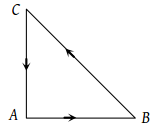• Subject:

...

• Topic:

...

Three forces starts acting simultaneously on a particle moving with velocity $\stackrel{\to }{v}.$ These forces are represented in magnitude and direction by the three sides of a triangle ABC (as shown). The particle will now move with velocity(1) $\stackrel{\to }{v}$ remaining unchanged

(2) Less than $\stackrel{\to }{v}$

(3) Greater than $\stackrel{\to }{v}$

(4) $\stackrel{\to }{v}$ in the direction of the largest force BC